Get instant live expert help with Excel or Google Sheets“My Excelchat expert helped me in less than 20 minutes, saving me what would have been 5 hours of work!”

#### Post your problem and you’ll get Expert help in seconds.

Your message must be at least 40 characters
Our professional Expert are available now. Your privacy is guaranteed.

# Learn How to Calculate Shipping Cost with VLOOKUP

We can use the VLOOKUP function to calculate the shipping cost for a specified weight of an item. We can also apply the MAX function to VLOOKUP in situations where we have a fixed minimum shipping cost no matter the weight.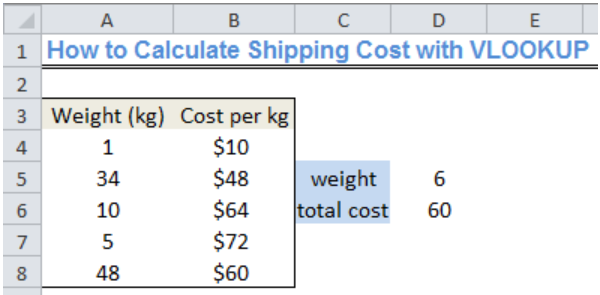Figure 1: Result of the VLOOKUP function for Calculating Shipping Cost

## Setting up the Data

• We will set up our data by inputting the weight of items and the corresponding cost per kilogram in Column A and Column B respectively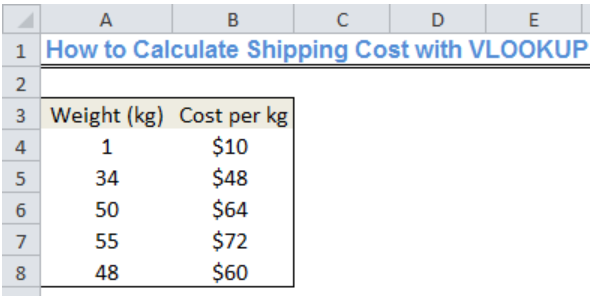Figure 2: Data for Calculating Shipping Cost with VLOOKUP

## Syntax for Calculating Shipping Costs using VLOOKUP

`=VLOOKUP(lookup_value,table_array,col_index_number,{range_lookup}* lookup_value`

## Explanation

• Lookup Value

This is the weight (the cell containing the weight) that we are interested in. For instance, if we want to find the cost of a 6 kg item, our lookup value will be will be 6

• Table Array

This is the range of values from where we will retrieve our value contained in the data. It is noteworthy that we must have at least two columns of data. Our range, in this case, is A4:B8.

• Column Index Number

This is the number of columns from where we will retrieve our data. In this case, we have just two columns; hence, our column index number is 2.

• Range Lookup

This will return values as TRUE or FALSE. TRUE (1) means that we want an approximate match and FALSE (2) implies that we want an exact match. We will opt for the approximate match because VLOOKUP is configured here by using the approximate match mode.

## Lookup Cost for 6kg

We will name a Cell that will contain the weight and have an empty cell where our formula will return our lookup value.

• Cell C5 will be called weight
• Cell D5 will contain the weight (6kg) whose total cost we intend to calculate
• Cell C6 will contain the total cost
• Cell D6 is where we will input our formula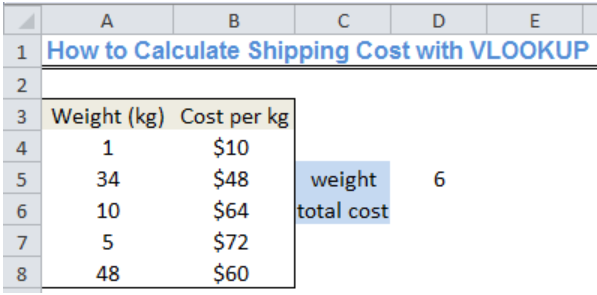Figure 3: Calculating Shipping Cost with VLOOKUP for 6kg

Based on the explanation of the syntax above, our formula is:

`=VLOOKUP(D5,A4:B8,2,1)*D5`

We will input this formula into Cell D6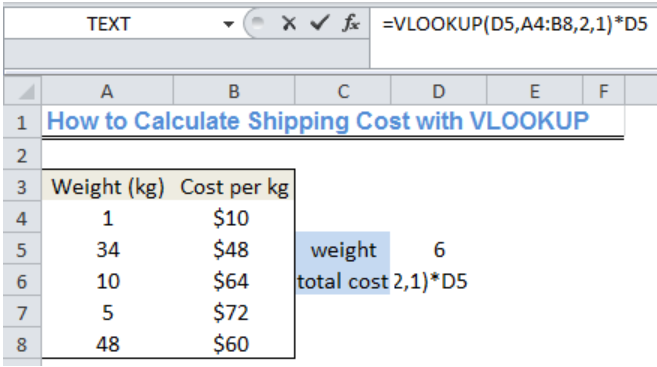Figure 4: Inputting the VLOOKUP formula

We will now press ENTER to get the result.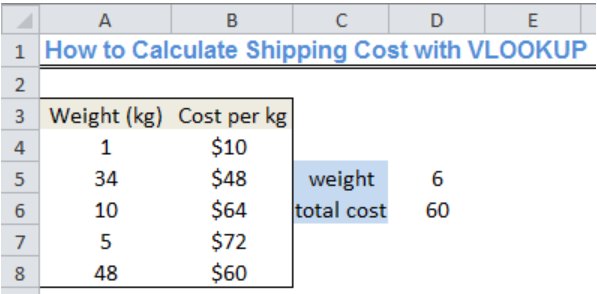Figure 5: Result of the VLOOKUP

The result has provided the amount for the total cost of shipping 6kg of an item.

## Note

The formula is set up such that it would return the value specified in the first row, which turns out to be slightly lesser than or equal to the value retrieved by the lookup value (the weight, 6kg). This simply means that the formula works based on the content of the first row (Cell A4 and Cell B4).

## Using the MAX function

We can use the MAX function for situations where there is a minimum fixed cost no matter the weight. We must remember that the least weight allowed for shipping is 1 kg as shown on the figures. Assuming the minimum fixed cost no matter the weight is \$40, the formula becomes this below:

`=MAX(VLOOKUP(D5,A4:B8,2,1)*D5,40)`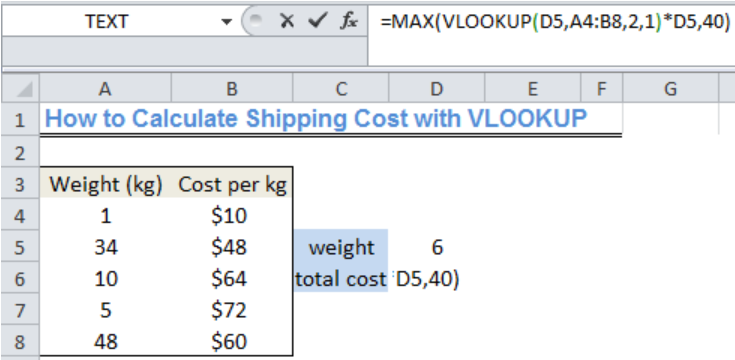Figure 6: Calculating Shipping Cost with VLOOKUP and MAX function

Press Enter after inserting the formula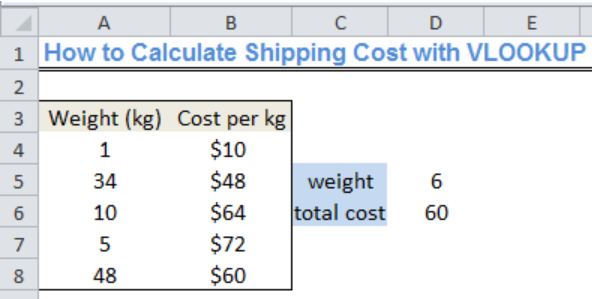Figure 7: Result of Shipping Cost with VLOOKUP and MAX function

If we change the weight to 1 kg in Cell D5, the total cost becomes \$40 because that is the least acceptable cost.

## Instant Connection to an Expert through our Excelchat Service

Most of the time, the problem you will need to solve will be more complex than a simple application of a formula or function. If you want to save hours of research and frustration, try our live Excelchat service! Our Excel Experts are available 24/7 to answer any Excel question you may have. We guarantee a connection within 30 seconds and a customized solution within 20 minutes.

Are you still looking for help with the VLOOKUP function? View our comprehensive round-up of VLOOKUP function tutorials here.

### Did this post not answer your question? Get a solution from connecting with the expert.Another blog reader asked this question today on Excelchat:
Solution examplesI filtered data in column A (it is labeled ID #) of a data set. On my next worksheet, column A is also the ID #, but it is a different data set. I want to filter the ID #'s the same for the two sheets, but how do I do that being that I am working with two different sets of data?
Solved by V. H. in 22 minsI'm looking for a formula or format for if there is data in c4 on a sheet... It adds extra information underneath
Solved by X. E. in 20 minshi, my vlookup wont work in some rows but does in others. its temperemental. e.g it will work on rows 10 to 15, then not row 16 and 17, but the will for the rest.
Solved by K. Q. in 21 minsUse the Vlookup Function to complete the "employee" column of table 2. Use "job Id" from table 2 as your lookup_value(s) and table 1 as your reference.
Solved by C. H. in 16 minsIf a cell in another sheet is populated I need a vlookup done. If the cell is not populated I need the cell to return blank.
Solved by T. D. in 60 mins## Subscribe to Excelchat.coAnother blog reader asked this question today on Excelchat: